﻿ BackgroundThe only one that I felt comfortable with was one that was infinite both in time and dimension (inward and outward). This gave me problems.

I never liked the idea of the Big Bang. It just never made any sense to me. But what was the alternative?

So light must decay. What does this mean? It can’t slow down; light travels at the speed of light (we only need to think of a vacuum here), so the decay must be a change in colour. The more energetic the light then the bigger the decay in any given time interval. This is good news for life.

This makes so much more sense to me than a universe whose edge is accelerating away from us (In every direction that’s explained by describing it as a 3D ‘surface’ on a 4D hypersphere, letting mathematics over-ride physics).

Black holes absorb matter, if they do that eternally, then they also have to emit matter (and I’m not talking about Hawking radiation here).

The big bang theory is underpinned by the following assumption.

• We know the colour of hydrogen. When we observe super-novae throughout the galaxy, we know from the colour shift in the hydrogen how fast it is travelling.
• When a sun goes supernova, it has a given energy signature. The brightness of this tells us how far it is away.

Next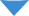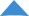NextNextIf it was infinite then black holes would ‘suck up’ all matter and the universe would be empty, they had all of eternity to do this, so it was inevitable. Even if this didn’t happen, the sky in an infinite universe would be white, as no matter where you looked, a star would be shining down on us. We would fry. So for this to work, my understanding of light and black holes had to change. Light already was problematic as the red shift caused by the expanding universe gave the age of the universe to be 13.8 Billion Years. But what if this was an illusion?

T

=

ln 2

½

0.693t

0.693

l

=

l

=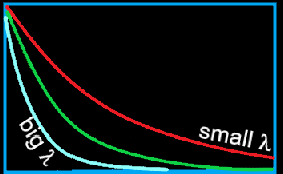Gamma and X-rays will decay the most. I imagine the decay follows similar principles to radioactive decay. A Gamma ray has a given chance of ‘losing’ its energy by interacting with matter between the source and us but some of it may be ‘lucky’ and arrive unaffected. Otherwise we would see no high energy radiation in the night sky.

This means that the red shift that is used to predict the age of the universe is an illusion. It also explains the observation that light at the ‘extreme edge’ of the universe is accelerating. The colour shift isn’t linear, instead it follows a logarithmic decay curve. The curve is steeper, the further the source is away from us giving the illusion of acceleration.

I can think only of two exceptions, see Black holes and Consciousness.

If we take this view, and I don’t, we no longer have to worry about the poor inhabitants of galaxies that are moving at significant fractions of the speed of light (Think of the kinetic energy involved in that!). More so, the galaxies that are now being found that are travelling faster than the speed of light are now much more well behaved. This isn’t to say that red shift does not exist but on balance, there is as much blue shift as red shift. Because of this, the decay curve is problematic as it measures two parameters, with the shift producing a wobble on the decay curve.Quasars are the biggest energy emitters in the universe. Physicists say they are related to supermassive black holes. I say they are black holes. So matter leaves the black hole at the poles. As matter cannot be emitted from a black hole, it must be ‘pushed’ out. Just as a pump cannot ‘suck’ water from a height greater than 10m (the point when a vacuum occurs), it can ‘push’ much greater heights than this. So the ‘matter’ inside a black hole must be organised so that it can push some of it across the event horizon (see for my attempt at describing this).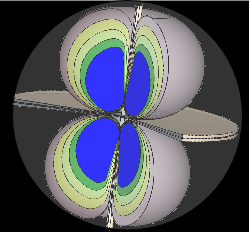To show how this works, let’s try it. Here is a table of velocities against measured distance (made up).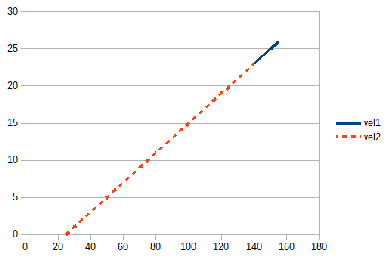The gradient is 26/(155-25) = 0.2 and the inverse of this is 5. The units are irrelevant but the age of this event is 5 units of time. Of course it depends on the assumption that the apparent velocity is the actual velocity of the measured item. If it isn’t then the assumption is nonsense. The apparent velocity of an emitting item is based on the frequency of the light being emitted. If the frequency is unchanging, this is easy but if the frequency changes (reduces), then the predictions fail.

So what if the photon ‘cools’ over time? It is possible to get the same apparent velocity if the frequency of the photon reduces over time. So an essentially static universe can appear to be expanding where the origin can be tracked back in time to the start. This would be an illusion.

There is only the ‘Is be’.

The ‘Was be’ and ‘Will be’ are constructions of the mind. It is an indicator that the consciousness does not follow the laws of nature. The mind does not obey the four known fundamental forces of nature. There is no room for free-will in either Quantum or Cosmic Physics. Just because it is imaginary does not mean it has no use. It is an essential parameter in our need to understand. We need it, the universe does not. Even when we throw the universe into a ‘wobble’ (extremely locally), it carries on regardless, taking our spontaneous interventions in its stride.